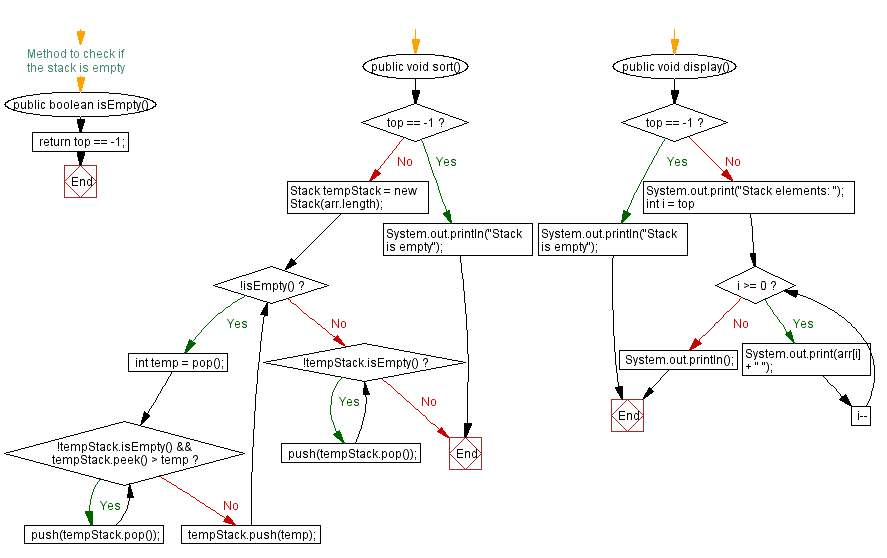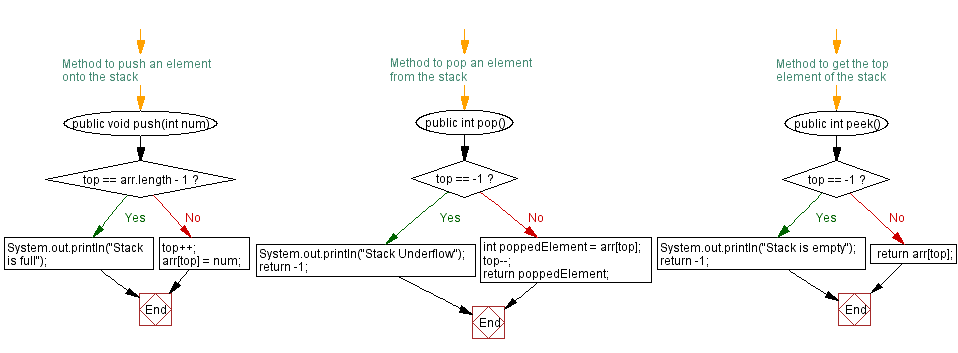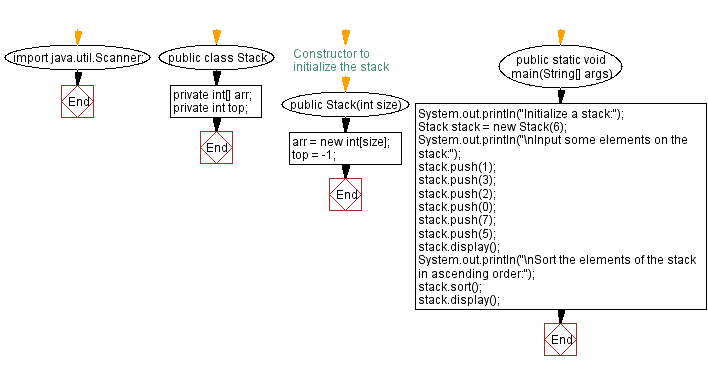# Java Exercises: Sort the elements of a stack in ascending order

## Java Stack: Exercise-2 with Solution

Write a Java program to sort the elements of a given stack in ascending order.

Sample Solution:

Java Code:

``````import java.util.Scanner;
public class Stack {
private int[] arr;
private int top;

// Constructor to initialize the stack
public Stack(int size) {
arr = new int[size];
top = -1;
}

// Method to push an element onto the stack
public void push(int num) {
if (top == arr.length - 1) {
System.out.println("Stack is full");
} else {
top++;
arr[top] = num;
}
}

// Method to pop an element from the stack
public int pop() {
if (top == -1) {
System.out.println("Stack Underflow");
return -1;
} else {
int poppedElement = arr[top];
top--;
return poppedElement;
}
}

// Method to get the top element of the stack
public int peek() {
if (top == -1) {
System.out.println("Stack is empty");
return -1;
} else {
return arr[top];
}
}

// Method to check if the stack is empty
public boolean isEmpty() {
}

public void sort() {
if (top == -1) {
System.out.println("Stack is empty");
return;
}

Stack tempStack = new Stack(arr.length);

while (!isEmpty()) {
int temp = pop();

while (!tempStack.isEmpty() && tempStack.peek() > temp) {
push(tempStack.pop());
}

tempStack.push(temp);
}

while (!tempStack.isEmpty()) {
push(tempStack.pop());
}
}

public void display() {
if (top == -1) {
System.out.println("Stack is empty");
} else {
System.out.print("Stack elements: ");
for (int i = top; i >= 0; i--) {
System.out.print(arr[i] + " ");
}
System.out.println();
}
}

public static void main(String[] args) {
System.out.println("Initialize a stack:");
Stack stack = new Stack(6);
System.out.println("\nInput some elements on the stack:");
stack.push(1);
stack.push(3);
stack.push(2);
stack.push(0);
stack.push(7);
stack.push(5);
stack.display();
System.out.println("\nSort the elements of the stack in ascending order:");
stack.sort();
stack.display();
}
}
```
```

Sample Output:

```Initialize a stack:

Input some elements on the stack:
Stack elements: 5 7 0 2 3 1

Sort the elements of the stack in ascending order:
Stack elements: 0 1 2 3 5 7
```

Flowchart:Live Demo:

Java Code Editor:

Improve this sample solution and post your code through Disqus

Java Stack Previous: Implement a stack with push and pop operations.
Java Stack Exercises Next: Sort the elements of a stack in descending order.

What is the difficulty level of this exercise?

Test your Programming skills with w3resource's quiz.

﻿